# Multiple integral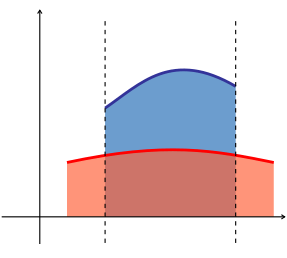Integral as area between two curves.Double integral as volume under a surface z = 10 − x2y2/8. The rectangular region at the bottom of the body is the domain of integration, while the surface is the graph of the two-variable function to be integrated.

The multiple integral is a generalization of the definite integral to functions of more than one real variable, for example, f(x, y) or f(x, y, z). Integrals of a function of two variables over a region in R2 are called double integrals, and integrals of a function of three variables over a region of R3 are called triple integrals.

## Introduction

Just as the definite integral of a positive function of one variable represents the area of the region between the graph of the function and the x-axis, the double integral of a positive function of two variables represents the volume of the region between the surface defined by the function (on the three-dimensional Cartesian plane where z = f(x, y)) and the plane which contains its domain. (The same volume can be obtained via the triple integral—the integral of a function in three variables—of the constant function f(x, y, z) = 1 over the above-mentioned region between the surface and the plane.) If there are more variables, a multiple integral will yield hypervolumes of multidimensional functions.

Multiple integration of a function in n variables: f(x1, x2, ..., xn) over a domain D is most commonly represented by nested integral signs in the reverse order of execution (the leftmost integral sign is computed last), followed by the function and integrand arguments in proper order (the integral with respect to the rightmost argument is computed last). The domain of integration is either represented symbolically for every argument over each integral sign, or is abbreviated by a variable at the rightmost integral sign:Since the concept of an antiderivative is only defined for functions of a single real variable, the usual definition of the indefinite integral does not immediately extend to the multiple integral.

## Mathematical definition

For n > 1, consider a so-called "half-open" n-dimensional hyperrectangular domain T, defined as:Partition each interval [aj, bj) into a finite family Ij of non-overlapping subintervals ijα, with each subinterval closed at the left end, and open at the right end.

Then the finite family of subrectangles C given byis a partition of T; that is, the subrectangles Ck are non-overlapping and their union is T.

Let f : TR be a function defined on T. Consider a partition C of T as defined above, such that C is a family of m subrectangles Cm andWe can approximate the total (n + 1)th-dimensional volume bounded below by the n-dimensional hyperrectangle T and above by the n-dimensional graph of f with the following Riemann sum:where Pk is a point in Ck and m(Ck) is the product of the lengths of the intervals whose Cartesian product is Ck, otherwise known as the measure of Ck.

The diameter of a subrectangle Ck is the largest of the lengths of the intervals whose Cartesian product is Ck. The diameter of a given partition of T is defined as the largest of the diameters of the subrectangles in the partition. Intuitively, as the diameter of the partition C is restricted smaller and smaller, the number of subrectangles m gets larger, and the measure m(Ck) of each subrectangle grows smaller. The function f is said to be Riemann integrable if the limitexists, where the limit is taken over all possible partitions of T of diameter at most δ.

If f is Riemann integrable, S is called the Riemann integral of f over T and is denotedFrequently this notation is abbreviated aswhere x represents the n-tuple (x1, ... xn) and dnx is the n-dimensional volume differential.

The Riemann integral of a function defined over an arbitrary bounded n-dimensional set can be defined by extending that function to a function defined over a half-open rectangle whose values are zero outside the domain of the original function. Then the integral of the original function over the original domain is defined to be the integral of the extended function over its rectangular domain, if it exists.

In what follows the Riemann integral in n dimensions will be called the multiple integral.

### Properties

Multiple integrals have many properties common to those of integrals of functions of one variable (linearity, commutativity, monotonicity, and so on). One important property of multiple integrals is that the value of an integral is independent of the order of integrands under certain conditions. This property is popularly known as Fubini's theorem.

### Particular cases

In the case of TR2, the integralis the double integral of f on T, and if TR3 the integralis the triple integral of f on T.

Notice that, by convention, the double integral has two integral signs, and the triple integral has three; this is a notational convention which is convenient when computing a multiple integral as an iterated integral, as shown later in this article.

## Methods of integration

The resolution of problems with multiple integrals consists, in most of cases, of finding a way to reduce the multiple integral to an iterated integral, a series of integrals of one variable, each being directly solvable. For continuous functions, this is justified by Fubini's theorem. Sometimes, it is possible to obtain the result of the integration by direct examination without any calculations.

The following are some simple methods of integration:

### Integrating constant functions

When the integrand is a constant function c, the integral is equal to the product of c and the measure of the domain of integration. If c = 1 and the domain is a subregion of R2, the integral gives the area of the region, while if the domain is a subregion of R3, the integral gives the volume of the region.

Example. Let f(x, y) = 2 andin which case,

since by definition we have:### Use of symmetry

When the domain of integration is symmetric about the origin with respect to at least one of the variables of integration and the integrand is odd with respect to this variable, the integral is equal to zero, as the integrals over the two halves of the domain have the same absolute value but opposite signs. When the integrand is even with respect to this variable, the integral is equal to twice the integral over one half of the domain, as the integrals over the two halves of the domain are equal.

Example 1. Consider the function f(x,y) = 2 sin(x) − 3y3 + 5 integrated over the domaina disc with radius 1 centered at the origin with the boundary included.

Using the linearity property, the integral can be decomposed into three pieces:The function 2 sin(x) is an odd function in the variable x and the disc T is symmetric with respect to the y-axis, so the value of the first integral is 0. Similarly, the function 3y3 is an odd function of y, and T is symmetric with respect to the x-axis, and so the only contribution to the final result is that of the third integral. Therefore the original integral is equal to the area of the disk times 5, or 5π.
Example 2. Consider the function f(x, y, z) = x exp(y2 + z2) and as integration region the sphere with radius 2 centered at the origin,The "ball" is symmetric about all three axes, but it is sufficient to integrate with respect to x-axis to show that the integral is 0, because the function is an odd function of that variable.

### Normal domains on R2

This method is applicable to any domain D for which:

• the projection of D onto either the x-axis or the y-axis is bounded by the two values, a and b
• any line perpendicular to this axis that passes between these two values intersects the domain in an interval whose endpoints are given by the graphs of two functions, α and β.

In all cases, the function to be integrated must be continuous on the domain.

#### x-axis

If the domain D is normal with respect to the x-axis, and f : DR is a continuous function; then α(x) and β(x) (defined on the interval [a, b]) are the two functions that determine D. Then:#### y-axis

If D is normal with respect to the y-axis and f : DR is a continuous function; then α(y) and β(y) (defined on the interval [a, b]) are the two functions that determine D. Then:#### Example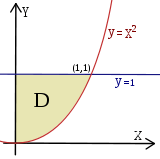Example: double integral over the normal region D

Consider the region (please see the graphic in the example):CalculateThis domain is normal with respect to both the x- and y-axes. To apply the formulae it is required to find the functions that determine D and the intervals over which these are defined. In this case the two functions are:while the interval is given by the intersections of the functions with x = 0, so the interval is [a, b] = [0, 1] (normality has been chosen with respect to the x-axis for a better visual understanding).

It is now possible to apply the formula:(at first the second integral is calculated considering x as a constant). The remaining operations consist of applying the basic techniques of integration:If we choose normality with respect to the y-axis we could calculateand obtain the same value.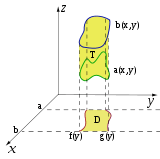Example of domain in R3 that is normal with respect to the xy-plane.

#### Normal domains on R3

The extension of these formulae to triple integrals should be apparent:

if T is a domain that is normal with respect to the xy-plane and determined by the functions α(x, y) and β(x, y), then(This definition is the same for the other five normality cases on R3.)

### Change of variables

The limits of integration are often not easily interchangeable (without normality or with complex formulae to integrate). One makes a change of variables to rewrite the integral in a more "comfortable" region, which can be described in simpler formulae. To do so, the function must be adapted to the new coordinates.

Example 1a. The function is f(x, y) = (x − 1)2 + y; if one adopts the substitution x′ = x − 1, y′ = y therefore x = x′ + 1, y = y one obtains the new function f2(x, y) = (x′)2 + y.
• Similarly for the domain because it is delimited by the original variables that were transformed before (x and y in example).
• the differentials dx and dy transform via the absolute value of the determinant of the Jacobian matrix containing the partial derivatives of the transformations regarding the new variable (consider, as an example, the differential transformation in polar coordinates).

There exist three main "kinds" of changes of variable (one in R2, two in R3); however, more general substitutions can be made using the same principle.

#### Polar coordinates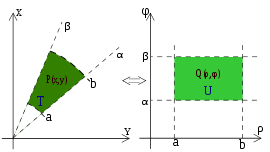Transformation from cartesian to polar coordinates.

In R2 if the domain has a circular symmetry and the function has some particular characteristics you can apply the transformation to polar coordinates (see the example in the picture) which means that the generic points P(x, y) in Cartesian coordinates switch to their respective points in polar coordinates. That allows one to change the shape of the domain and simplify the operations.

The fundamental relation to make the transformation is the following:Example 2a. The function is f(x, y) = x + y and applying the transformation one obtainsExample 2b. The function is f(x, y) = x2 + y2, in this case one has:using the Pythagorean trigonometric identity (very useful to simplify this operation).

The transformation of the domain is made by defining the radius' crown length and the amplitude of the described angle to define the ρ, φ intervals starting from x, y.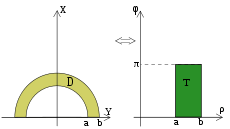Example of a domain transformation from cartesian to polar.
Example 2c. The domain is D = {x2 + y2 ≤ 4}, that is a circumference of radius 2; it's evident that the covered angle is the circle angle, so φ varies from 0 to 2π, while the crown radius varies from 0 to 2 (the crown with the inside radius null is just a circle).
Example 2d. The domain is D = {x2 + y2 ≤ 9, x2 + y2 ≥ 4, y ≥ 0}, that is the circular crown in the positive y half-plane (please see the picture in the example); φ describes a plane angle while ρ varies from 2 to 3. Therefore the transformed domain will be the following rectangle:The Jacobian determinant of that transformation is the following:which has been obtained by inserting the partial derivatives of x = ρ cos(φ), y = ρ sin(φ) in the first column respect to ρ and in the second respect to φ, so the dx dy differentials in this transformation become ρ dρ dφ.

Once the function is transformed and the domain evaluated, it is possible to define the formula for the change of variables in polar coordinates:φ is valid in the [0, 2π] interval while ρ, which is a measure of a length, can only have positive values.
Example 2e. The function is f(x, y) = x and the domain is the same as in Example 2d. From the previous analysis of D we know the intervals of ρ (from 2 to 3) and of φ (from 0 to π). Now we change the function:finally let's apply the integration formula:Once the intervals are known, you have#### Cylindrical coordinates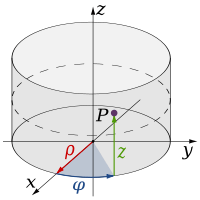Cylindrical coordinates.

In R3 the integration on domains with a circular base can be made by the passage to cylindrical coordinates; the transformation of the function is made by the following relation:The domain transformation can be graphically attained, because only the shape of the base varies, while the height follows the shape of the starting region.

Example 3a. The region is D = {x2 + y2 ≤ 9, x2 + y2 ≥ 4, 0 ≤ z ≤ 5} (that is the "tube" whose base is the circular crown of Example 2d and whose height is 5); if the transformation is applied, this region is obtained:(that is, the parallelepiped whose base is similar to the rectangle in Example 2d and whose height is 5).

Because the z component is unvaried during the transformation, the dx dy dz differentials vary as in the passage to polar coordinates: therefore, they become ρ dρ dφ dz.

Finally, it is possible to apply the final formula to cylindrical coordinates:This method is convenient in case of cylindrical or conical domains or in regions where it is easy to individuate the z interval and even transform the circular base and the function.
Example 3b. The function is f(x, y, z) = x2 + y2 + z and as integration domain this cylinder: D = {x2 + y2 ≤ 9, −5 ≤ z ≤ 5 }. The transformation of D in cylindrical coordinates is the following:while the function becomesFinally one can apply the integration formula:developing the formula you have#### Spherical coordinates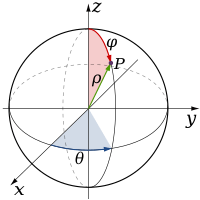Spherical coordinates.

In R3 some domains have a spherical symmetry, so it's possible to specify the coordinates of every point of the integration region by two angles and one distance. It's possible to use therefore the passage to spherical coordinates; the function is transformed by this relation:Points on the z-axis do not have a precise characterization in spherical coordinates, so θ can vary between 0 and 2π.

The better integration domain for this passage is obviously the sphere.

Example 4a. The domain is D = x2 + y2 + z2 ≤ 16 (sphere with radius 4 and center at the origin); applying the transformation you get the regionThe Jacobian determinant of this transformation is the following:The dx dy dz differentials therefore are transformed to ρ2 sin(φ) .

This yields the final integration formula:It is better to use this method in case of spherical domains and in case of functions that can be easily simplified by the first fundamental relation of trigonometry extended to R3 (see Example 4b); in other cases it can be better to use cylindrical coordinates (see Example 4c).The extra ρ2 and sin φ come from the Jacobian.

In the following examples the roles of φ and θ have been reversed.

Example 4b. D is the same region as in Example 4a and f(x, y, z) = x2 + y2 + z2 is the function to integrate. Its transformation is very easy:while we know the intervals of the transformed region T from D:We therefore apply the integration formula:and, developing, we getExample 4c. The domain D is the ball with center at the origin and radius 3a,and f(x, y, z) = x2 + y2 is the function to integrate.

Looking at the domain, it seems convenient to adopt the passage to spherical coordinates, in fact, the intervals of the variables that delimit the new T region are obviously:However, applying the transformation, we get.

Applying the formula for integration we obtain:which is very hard to solve. This problem will be solved by using the passage to cylindrical coordinates. The new T intervals arethe z interval has been obtained by dividing the ball into two hemispheres simply by solving the inequality from the formula of D (and then directly transforming x2 + y2 into ρ2). The new function is simply ρ2. Applying the integration formula.

Then we getThanks to the passage to cylindrical coordinates it was possible to reduce the triple integral to an easier one-variable integral.

See also the differential volume entry in nabla in cylindrical and spherical coordinates.

## Examples

### Double integral

Let us assume that we wish to integrate a multivariable function f over a region A:From this we formulate the double integralThe inner integral is performed first, integrating with respect to x and taking y as a constant, as it is not the variable of integration. The result of this integral, which is a function depending only on y, is then integrated with respect to y.We then integrate the result with respect to y.Observe that the order of integration is sometimes interchangeable:The instances where the order is interchangeable is determined by Fubini's theorem.

### Computing a volume

Using the methods previously described, it is possible to calculate the volumes of some common solids.

• Cylinder: The volume of a cylinder with height h and circular base of radius R can be calculated by integrating the constant function h over the circular base, using polar coordinates.This is in agreement with the formula for the volume of a prism.
• Sphere: The volume of a sphere with radius R can be calculated by integrating the constant function 1 over the sphere, using spherical coordinates.• Tetrahedron (triangular pyramid or 3-simplex): The volume of a tetrahedron with its apex at the origin and edges of length l along the x-, y- and z-axes can be calculated by integrating the constant function 1 over the tetrahedron.This is in agreement with the formula for the volume of a pyramid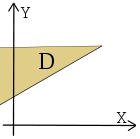Example of an improper domain.

## Multiple improper integral

In case of unbounded domains or functions not bounded near the boundary of the domain, we have to introduce the double improper integral or the triple improper integral.

## Multiple integrals and iterated integrals

Fubini's theorem states that ifthat is, if the integral is absolutely convergent, then the multiple integral will give the same result as the iterated integral,In particular this will occur if |f(x, y)| is a bounded function and A and B are bounded sets.

If the integral is not absolutely convergent, care is needed not to confuse the concepts of multiple integral and iterated integral, especially since the same notation is often used for either concept. The notationmeans, in some cases, an iterated integral rather than a true double integral. In an iterated integral, the outer integralis the integral with respect to x of the following function of x:A double integral, on the other hand, is defined with respect to area in the xy-plane. If the double integral exists, then it is equal to each of the two iterated integrals (either "dy dx" or "dx dy") and one often computes it by computing either of the iterated integrals. But sometimes the two iterated integrals exist when the double integral does not, and in some such cases the two iterated integrals are different numbers, i.e., one hasThis is an instance of rearrangement of a conditionally convergent integral.

On the other hand, some conditions ensure that the two iterated integrals are equal even though the double integral need not exist. By the FichtenholzLichtenstein theorem, if f is bounded on [0, 1] × [0, 1] and both iterated integrals exist, then they are equal. Moreover, existence of the inner integrals ensures existence of the outer integrals. The double integral need not exist in this case even as Lebesgue integral, according to Sierpiński.

The notationmay be used if one wishes to be emphatic about intending a double integral rather than an iterated integral.

## Some practical applications

Quite generally, just as in one variable, one can use the multiple integral to find the average of a function over a given set. Given a set DRn and an integrable function f over D, the average value of f over its domain is given bywhere m(D) is the measure of D.

Additionally, multiple integrals are used in many applications in physics. The examples below also show some variations in the notation.

In mechanics, the moment of inertia is calculated as the volume integral (triple integral) of the density weighed with the square of the distance from the axis:The gravitational potential associated with a mass distribution given by a mass measure dm on three-dimensional Euclidean space R3 isIf there is a continuous function ρ(x) representing the density of the distribution at x, so that dm(x) = ρ(x)d3x, where d3x is the Euclidean volume element, then the gravitational potential isIn electromagnetism, Maxwell's equations can be written using multiple integrals to calculate the total magnetic and electric fields. In the following example, the electric field produced by a distribution of charges given by the volume charge density ρ( r ) is obtained by a triple integral of a vector function:This can also be written as an integral with respect to a signed measure representing the charge distribution.

• Main analysis theorems that relate multiple integrals:

## References

1. Stewart, James (2008). Calculus: Early Transcendentals (6th ed.). Brooks Cole Cengage Learning. ISBN 978-0-495-01166-8.
2. Larson; Edwards (2014). Multivariable Calculus (10th ed.). Cengage Learning. ISBN 978-1-285-08575-3.
3. Rudin, Walter. Principles of Mathematical Analysis. Walter Rudin Student Series in Advanced Mathematics (3rd ed.). McGraw–Hill. ISBN 978-0-07-054235-8.
4. Jones, Frank (2001). Lebesgue Integration on Euclidean Space. Jones and Bartlett. pp. 527–529.
5. Lewin, Jonathan (2003). An interactive introduction to mathematical analysis. Cambridge. Sect. 16.6.
6. Lewin, Jonathan (1987). "Some applications of the bounded convergence theorem for an introductory course in analysis". The American Mathematical Monthly. AMS. 94 (10): 988–993. doi:10.2307/2322609.
7. Sinclair, George Edward (1974). "A finitely additive generalization of the Fichtenholz–Lichtenstein theorem". Transactions of the American Mathematical Society. AMS. 193: 359–374. doi:10.2307/1996919.
8. Bogachev, Vladimir I. (2006). Measure Theory. 1. Springer. Item 3.10.49.
9. Kibble, Tom W. B.; Berkshire, Frank H. (2004). Classical Mechanics (5th ed.). Imperial College Press. ISBN 978-1-86094-424-6.
10. Jackson, John D. (1998). Classical Electrodynamics (3rd ed.). Wiley. ISBN 0-471-30932-X.